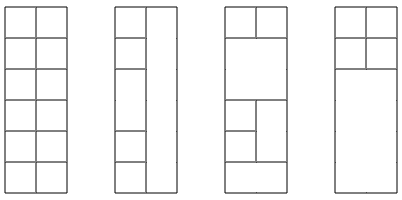CSES - Counting Towers
• Time limit: 1.00 s
• Memory limit: 512 MB

Your task is to build a tower whose width is 2 and height is n. You have an unlimited supply of blocks whose width and height are integers.

For example, here are some possible solutions for n=6:Given n, how many different towers can you build? Mirrored and rotated towers are counted separately if they look different.

# Input

The first input line contains an integer t: the number of tests.

After this, there are t lines, and each line contains an integer n: the height of the tower.

# Output

For each test, print the number of towers modulo 10^9+7.

# Constraints

• 1 \le t \le 100
• 1 \le n \le 10^6

# Example

Input:

3
2
6
1337


Output:

8
2864
640403945